+关注继续查看

# 第2章 构造数据抽象

--Hermann Weyl，The Mathematical Way of Thinking
（思维的数学方式）

(define (linear-combination a b x y)
(+ (* a x) (* b y)))

(define (linear-combination a b x y)
(add (mul a x) (mul b y)))

## 2.1 数据抽象导引

### 2.1.1 实例：有理数的算术运算

• (make-rat ）返回一个有理数，其分子是整数，分母是整数。
• (numer ）返回有理数的分子。
• (denom ）返回有理数的分母。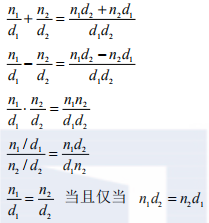(define (add-rat x y)
(make-rat (+ (* (numer x) (denom y))
(* (numer y) (denom x)))
(* (denom x) (denom y))))
(define (sub-rat x y)
(make-rat (- (* (numer x) (denom y))
(* (numer y) (denom x)))
(* (denom x) (denom y))))
(define (mul-rat x y)
(make-rat (* (numer x) (numer y))
(* (denom x) (denom y))))
(define (div-rat x y)
(make-rat (* (numer x) (denom y))
(* (denom x) (numer y))))
(define (equal-rat? x y)
(= (* (numer x) (denom y))
(* (numer y) (denom x))))

(define x (cons 1 2))
(car x)
1
(cdr x)
2

(define x (cons 1 2))
(define y (cons 3 4))
(define z (cons x y))
(car (car z))
1
(car (cdr z))
3

(define (make-rat n d) (cons n d))
(define (numer x) (car x))
(define (denom x) (cdr x))

(define (print-rat x)
(newline)
(display (numer x))
(display "/")
(display (denom x)))

(define one-half (make-rat 1 2))
(print-rat one-half)
1/2
(define one-third (make-rat 1 3))
5/6
(print-rat (mul-rat one-half one-third))
1/6
6/9

(define (make-rat n d)
(let ((g (gcd n d)))
(cons (/ n g) (/ d g))))

(print-rat (add-rat one-third one-third))
2/3


### 2.1.2 抽象屏障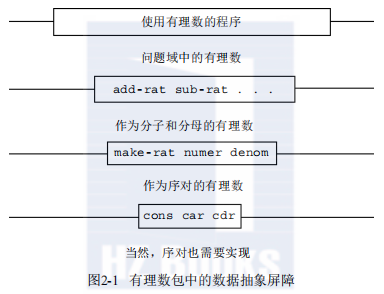(define (make-rat n d)
(cons n d))
(define (numer x)
(let ((g (gcd (car x) (cdr x))))
(/ (car x) g)))
(define (denom x)
(let ((g (gcd (car x) (cdr x))))
(/ (cdr x) g)))

(define (print-point p)
(newline)
(display "(")
(display (x-point p))
(display ",")
(display (y-point p))
(display ")"))

### 2.1.3 数据意味着什么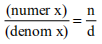(define (cons x y)
(define (dispatch m)
(cond ((= m 0) x)
((= m 1) y)
(else (error "Argument not 0 or 1 -- CONS" m))))
dispatch)
(define (car z) (z 0))
(define (cdr z) (z 1))

(define (cons x y)
(lambda (m) (m x y)))
(define (car z)
(z (lambda (p q) p)))

(define zero (lambda (f) (lambda (x) x)))
(lambda (f) (lambda (x) (f ((n f) x)))))

### 2.1.4 扩展练习：区间算术

Alyssa P. Hacker正在设计一个帮助人们求解工程问题的系统。她希望这个系统提供的一个特征是能够去操作不准确的量（例如物理设备的测量参数），这种量具有已知的精度，所以，在对这种近似量进行计算时，得到的结果也应该是已知精度的数值。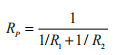Alyssa的想法是实现一套“区间算术”，即作为可以用于组合“区间”（表示某种不准确量的可能值的对象）的一组算术运算。两个区间的加、减、乘、除的结果仍是一个区间，表示的是计算结果的范围。
Alyssa假设有一种称为“区间”的抽象对象，这种对象有两个端点，一个下界和一个上界。她还假定，给了一个区间的两个端点，就可以用数据构造函数make-interval构造出相应的区间来。Alyssa首先写出了一个做区间加法的过程，她推理说，和的最小值应该是两个区间的下界之和，其最大值应该是两个区间的上界之和：

(define (add-interval x y)
(make-interval (+ (lower-bound x) (lower-bound y))
(+ (upper-bound x) (upper-bound y))))

Alyssa还找出了这种界的乘积的最小和最大值，用它们做出了两个区间的乘积（min和max是求出任意多个参数中的最小值和最大值的基本过程）。

(define (mul-interval x y)
(let ((p1 (* (lower-bound x) (lower-bound y)))
(p2 (* (lower-bound x) (upper-bound y)))
(p3 (* (upper-bound x) (lower-bound y)))
(p4 (* (upper-bound x) (upper-bound y))))
(make-interval (min p1 p2 p3 p4)
(max p1 p2 p3 p4))))

(define (div-interval x y)
(mul-interval x
(make-interval (/ 1.0 (upper-bound y))
(/ 1.0 (lower-bound y)))))

(define (make-interval a b) (cons a b))

(define (make-center-width c w)
(make-interval (- c w) (+ c w)))
(define (center i)
(/ (+ (lower-bound i) (upper-bound i)) 2))
(define (width i)
(/ (- (upper-bound i) (lower-bound i)) 2))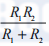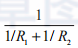(define (par1 r1 r2)
(div-interval (mul-interval r1 r2)
(define (par2 r1 r2)
(let ((one (make-interval 1 1)))
(div-interval one
(div-interval one r2)))))

Lem抱怨说，Alyssa程序对两种不同计算方法给出不同的值。这确实是很严重的抱怨。

## 2.2 层次性数据和闭包性质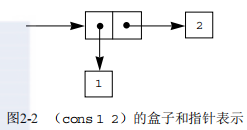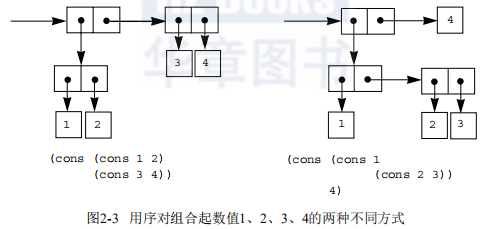### 2.2.1 序列的表示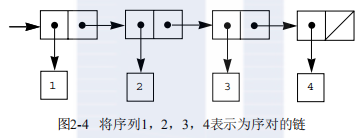(cons 1
(cons 2
(cons 3
(cons 4 nil))))

(list <a1> <a2> ... <an>)

(cons <a1> (cons <a2> (cons ... (cons <an> nil) ...)))

Lisp系统通常用元素序列的形式打印出表，外面用括号括起。按照这种方式，图2-4里的数据对象就将打印为（1 2 3 4）：

(define one-through-four (list 1 2 3 4))
one-through-four
(1 2 3 4)

(car one-through-four)
1
(cdr one-through-four)
(2 3 4)
(car (cdr one-through-four))
2
(cons 10 one-through-four)
(10 1 2 3 4)
(cons 5 one-through-four)
(5 1 2 3 4)

nil的值用于表示序对的链结束，它也可以当作一个不包含任何元素的序列，空表。单词“nil”是拉丁词汇“nihil”的缩写，这个拉丁词汇表示“什么也没有”。

• 对n＝0，list-ref应返回表的car。
• 否则，list-ref返回表的cdr的第（n－1）个项。
(define (list-ref items n)
(if (= n 0)
(car items)
(list-ref (cdr items) (- n 1))))
(define squares (list 1 4 9 16 25))
(list-ref squares 3)
16

(define (length items)
(if (null? items)
0
(+ 1 (length (cdr items)))))
(define odds (list 1 3 5 7))
(length odds)
4

• 任意一个表的length就是这个表的cdr的length加一。

• 空表的length是0。

(define (length items)
(define (length-iter a count)
(if (null? a)
count
(length-iter (cdr a) (+ 1 count))))
(length-iter items 0))

(append squares odds)
(1 4 9 16 25 1 3 5 7)
(append odds squares)
(1 3 5 7 1 4 9 16 25)

append也是用一种递归方案实现的。要得到表list1和list2的append，按如下方式做：

• 如果list1是空表，结果就是list2。
• 否则应先做出list1的cdr和list2的append，而后再将list1的car通过cons加到结果的前面：
(define (append list1 list2)
(if (null? list1)
list2
(cons (car list1) (append (cdr list1) list2))))

(last-pair (list 23 72 149 34))
(34)

(reverse (list 1 4 9 16 25))
(25 16 9 4 1)

(define us-coins (list 50 25 10 5 1))
(define uk-coins (list 100 50 20 10 5 2 1 0.5))

(cc 100 us-coins)
292

(define (cc amount coin-values)
(cond ((= amount 0) 1)
((or (< amount 0) (no-more? coin-values)) 0)
(else
(+ (cc amount
(except-first-denomination coin-values))
(cc (- amount
(first-denomination coin-values))
coin-values)))))

(define (f x y . z) <body>)

(f 1 2 3 4 5 6)

(define (g . w) <body>)

(g 1 2 3 4 5 6)

(same-parity 1 2 3 4 5 6 7)
(1 3 5 7)
(same-parity 2 3 4 5 6 7)
(2 4 6)

(define (scale-list items factor)
(if (null? items)
nil
(cons (* (car items) factor)
(scale-list (cdr items) factor))))
(scale-list (list 1 2 3 4 5) 10)
(10 20 30 40 50)

(define (map proc items)
(if (null? items)
nil
(cons (proc (car items))
(map proc (cdr items)))))
(map abs (list -10 2.5 -11.6 17))
(10 2.5 11.6 17)
(map (lambda (x) (* x x))
(list 1 2 3 4))
(1 4 9 16)

(define (scale-list items factor)
(map (lambda (x) (* x factor))
items))

map是一种很重要的结构，不仅因为它代表了一种公共模式，而且因为它建立起了一种处理表的高层抽象。在scale-list原来的定义里，程序的递归结构将人的注意力吸引到对于表中逐个元素的处理上。通过map定义scale-list抑制了这种细节层面上的情况，强调的是从元素表到结果表的一个缩放变换。这两种定义形式之间的差异，并不在于计算机会执行不同的计算过程（其实不会），而在于我们对这同一个过程的不同思考方式。从作用上看，map帮我们建起了一层抽象屏障，将实现表变换的过程的实现，与如何提取表中元素以及组合结果的细节隔离开。与图2-1里所示的屏障类似，这种抽象也提供了新的灵活性，使我们有可能在保持从序列到序列的变换操作框架的同时，改变序列实现的低层细节。2.2.3节将把序列的这种使用方式扩展为一种组织程序的框架。

(square-list (list 1 2 3 4))
(1 4 9 16)

(define (square-list items)
(if (null? items)
nil
(cons <??> <??>)))
(define (square-list items)
(map <??> <??>))

(define (square-list items)
(if (null? things)
(iter (cdr things)
(cons (square (car things))
(iter items nil))

Louis又试着修正其程序，交换了cons的参数：

(define (square-list items)
(if (null? things)
(iter (cdr things)
(square (car things))))))
(iter items nil))

(for-each (lambda (x) (newline) (display x))
(list 57 321 88))
57
321
88

### 2.2.2 层次性结构

(cons (list 1 2) (list 3 4))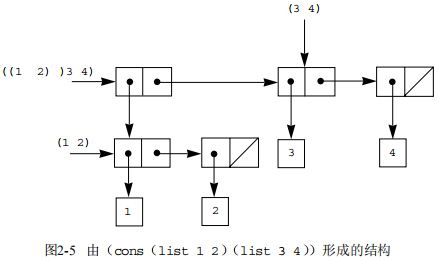(define x (cons (list 1 2) (list 3 4)))
(length x)
3
(count-leaves x)
4
(list x x)
(((1 2) 3 4) ((1 2) 3 4))
(length (list x x))
2
(count-leaves (list x x))
8

• 表x的length是x的cdr的length加一。
• 空表的length是0。

count-leaves的递归方案与此类似，对于空表的值也相同：

• 空表的count-leaves是0，

• 对于树x的count-leaves应该是x的car的count-leaves与x的cdr的count-leaves之和。

• 一个树叶的count-leaves是1。

(define (count-leaves x)
(cond ((null? x) 0)
((not (pair? x)) 1)
(else (+ (count-leaves (car x))
(count-leaves (cdr x))))))

(1 3 (5 7) 9)
((7))
(1 (2 (3 (4 (5 (6 7))))))

(define x (list 1 2 3))
(define y (list 4 5 6))

(append x y)
(cons x y)
(list x y)

(define x (list (list 1 2) (list 3 4)))
x
((1 2) (3 4))
(reverse x)
((3 4) (1 2))
(deep-reverse x)
((4 3) (2 1))

(define x (list (list 1 2) (list 3 4)))
(fringe x)
(1 2 3 4)
(fringe (list x x))
(1 2 3 4 1 2 3 4)

(define (make-mobile left right)
(list left right))

(define (make-branch length structure)
(list length structure))

a) 请写出相应的选择函数left-branch和right-branch，它们分别返回活动体的两个分支。还有branch-length和branch-structure，它们返回一个分支上的成分。
b) 用你的选择函数定义过程total-weight，它返回一个活动体的总重量。
c) 一个活动体称为是平衡的，如果其左分支的力矩等于其右分支的力矩（也就是说，如果其左杆的长度乘以吊在杆上的重量，等于这个活动体右边的同样乘积），而且在其每个分支上吊着的子活动体也都平衡。请设计一个过程，它能检查一个二叉活动体是否平衡。
d) 假定我们改变活动体的表示，采用下面构造方式：

(define (make-mobile left right)
(cons left right))
(define (make-branch length structure)
(cons length structure))

map是处理序列的一种强有力抽象，与此类似，map与递归的结合也是处理树的一种强有力抽象。举例来说，可以有与2.2.1节的scale-list类似的scale-tree过程，以一个数值因子和一棵叶子为数值的树作为参数，返回一棵具有同样形状的树，树中的每个数值都乘以了这个因子。对于scale-tree的递归方案也与count-leaves的类似：

(define (scale-tree tree factor)
(cond ((null? tree) nil)
((not (pair? tree)) (* tree factor))
(else (cons (scale-tree (car tree) factor)
(scale-tree (cdr tree) factor)))))
(scale-tree (list 1 (list 2 (list 3 4) 5) (list 6 7))
10)
(10 (20 (30 40) 50) (60 70))

(define (scale-tree tree factor)
(map (lambda (sub-tree)
(if (pair? sub-tree)
(scale-tree sub-tree factor)
(* sub-tree factor)))
tree))

(square-tree
(list 1
(list 2 (list 3 4) 5)
(list 6 7)))
(1 (4 (9 16) 25) (36 49))

(define (square-tree tree) (tree-map square tree))

(define (subsets s)
(if (null? s)
(list nil)
(let ((rest (subsets (cdr s))))
(append rest (map <??> rest)))))

### 2.2.3 序列作为一种约定的界面

(define (sum-odd-squares tree)
(cond ((null? tree) 0)
((not (pair? tree))
(if (odd? tree) (square tree) 0))
(else (+ (sum-odd-squares (car tree))
(sum-odd-squares (cdr tree))))))

(define (even-fibs n)
(define (next k)
(if (> k n)
nil
(let ((f (fib k)))
(if (even? f)
(cons f (next (+ k 1)))
(next (+ k 1))))))
(next 0))

• 枚举出一棵树的树叶；
• 过滤它们，选出其中的奇数；
• 对选出的每一个数求平方；
• 用＋累积起得到的结果，从0开始。

• 枚举从0到n的整数；
• 对每个整数计算相应的斐波那契数；
• 过滤它们，选出其中的偶数；
• 用cons累积得到的结果，从空表开始。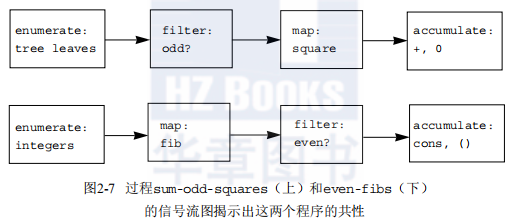(map square (list 1 2 3 4 5))
(1 4 9 16 25)

(define (filter predicate sequence)
(cond ((null? sequence) nil)
((predicate (car sequence))
(cons (car sequence)
(filter predicate (cdr sequence))))
(else (filter predicate (cdr sequence)))))

(filter odd? (list 1 2 3 4 5))
(1 3 5)

(define (accumulate op initial sequence)
(if (null? sequence)
initial
(op (car sequence)
(accumulate op initial (cdr sequence)))))
(accumulate + 0 (list 1 2 3 4 5))
15
(accumulate * 1 (list 1 2 3 4 5))
120
(accumulate cons nil (list 1 2 3 4 5))
(1 2 3 4 5)

(define (enumerate-interval low high)
(if (> low high)
nil
(cons low (enumerate-interval (+ low 1) high))))
(enumerate-interval 2 7)
(2 3 4 5 6 7)

(define (enumerate-tree tree)
(cond ((null? tree) nil)
((not (pair? tree)) (list tree))
(else (append (enumerate-tree (car tree))
(enumerate-tree (cdr tree))))))
(enumerate-tree (list 1 (list 2 (list 3 4)) 5))
(1 2 3 4 5)

(define (sum-odd-squares tree)
(accumulate +
0
(map square
(filter odd?
(enumerate-tree tree)))))

(define (even-fibs n)
(accumulate cons
nil
(filter even?
(map fib
(enumerate-interval 0 n)))))

(define (list-fib-squares n)
(accumulate cons
nil
(map square
(map fib
(enumerate-interval 0 n)))))
(list-fib-squares 10)
(0 1 1 4 9 25 64 169 441 1156 3025)

(define (product-of-squares-of-odd-elements sequence)
(accumulate *
1
(map square
(filter odd? sequence))))
(product-of-squares-of-odd-elements (list 1 2 3 4 5))
225

(define (salary-of-highest-paid-programmer records)
(accumulate max
0
(map salary
(filter programmer? records))))

(define (map p sequence)
(accumulate (lambda (x y) <??>) nil sequence))
(define (append seq1 seq2)
(accumulate cons <??> <??>))
(define (length sequence)
(accumulate <??> 0 sequence))

anxn＋an－1xn－1＋…＋a1x＋a0

(… (anx＋an－1) x＋…＋a1) x＋a0

(define (horner-eval x coefficient-sequence)
(accumulate (lambda (this-coeff higher-terms) <??>)
0
coefficient-sequence))

(horner-eval 2 (list 1 3 0 5 0 1))

(define (count-leaves t)
(accumulate <??> <??> (map <??> <??>)))

(define (accumulate-n op init seqs)
(if (null? (car seqs))
nil
(cons (accumulate op init <??>)
(accumulate-n op init <??>))))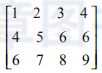(dot-product v w)      返回和穒viwi;
(matrix-*-vector m v)  返回向量 t，其中ti＝穓mijvj;
(matrix-*-matrix m n)  返回矩阵 p，其中pij＝穔miknkj;
(transpose m)          返回矩阵 n，其中nij＝mji.

(define (dot-product v w)
(accumulate + 0 (map * v w)))

(define (matrix-*-vector m v)
(map <??> m))
(define (transpose mat)
(accumulate-n <??> <??> mat))
(define (matrix-*-matrix m n)
(let ((cols (transpose n)))
(map <??> m)))

(define (fold-left op initial sequence)
(define (iter result rest)
(if (null? rest)
result
(iter (op result (car rest))
(cdr rest))))
(iter initial sequence))

(fold-right / 1 (list 1 2 3))
(fold-left / 1 (list 1 2 3))
(fold-right list nil (list 1 2 3))
(fold-left list nil (list 1 2 3))

(define (reverse sequence)
(fold-right (lambda (x y) <??>) nil sequence))
(define (reverse sequence)
(fold-left (lambda (x y) <??>) nil sequence))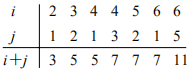(accumulate append
nil
(map (lambda (i)
(map (lambda (j) (list i j))
(enumerate-interval 1 (- i 1))))
(enumerate-interval 1 n)))

(define (flatmap proc seq)
(accumulate append nil (map proc seq)))

(define (prime-sum? pair)
(prime? (+ (car pair) (cadr pair))))

(define (make-pair-sum pair)
(list (car pair) (cadr pair) (+ (car pair) (cadr pair))))

(define (prime-sum-pairs n)
(map make-pair-sum
(filter prime-sum?
(flatmap
(lambda (i)
(map (lambda (j) (list i j))
(enumerate-interval 1 (- i 1))))
(enumerate-interval 1 n)))))

(define (permutations s)
(if (null? s)                    ; empty set?
(list nil)                   ; sequence containing empty set
(flatmap (lambda (x)
(map (lambda (p) (cons x p))
(permutations (remove x s))))
s)))

(define (remove item sequence)
(filter (lambda (x) (not (= x item)))
sequence))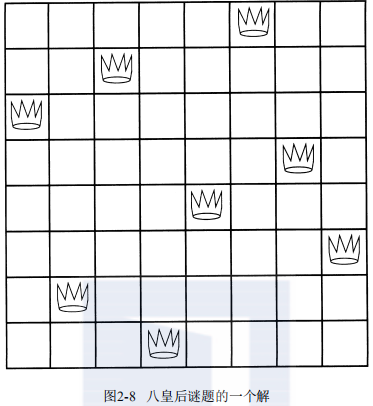(define (queens board-size)
(define (queen-cols k)
(if (= k 0)
(list empty-board)
(filter
(lambda (positions) (safe? k positions))
(flatmap
(lambda (rest-of-queens)
(map (lambda (new-row)
(enumerate-interval 1 board-size)))
(queen-cols (- k 1))))))
(queen-cols board-size))

(flatmap
(lambda (new-row)
(map (lambda (rest-of-queens)
(queen-cols (- k 1))))
(enumerate-interval 1 board-size))

### 2.2.4 实例：一个图形语言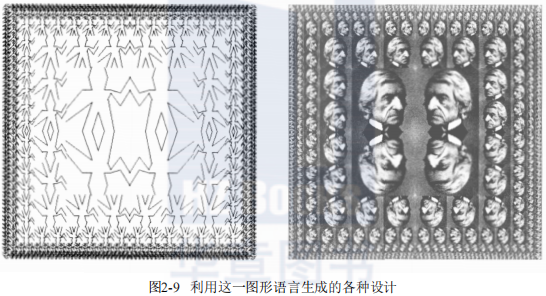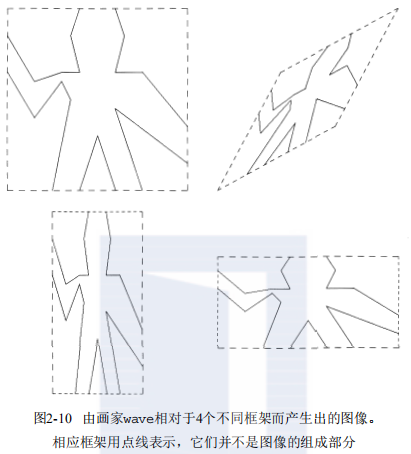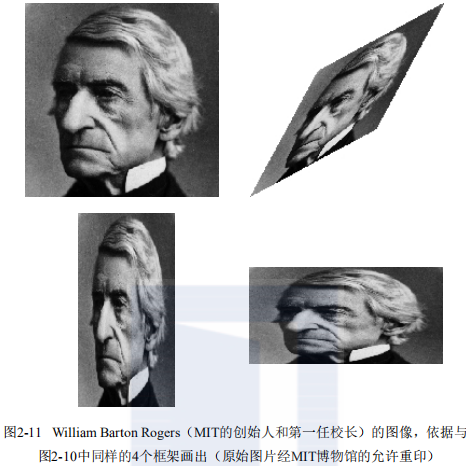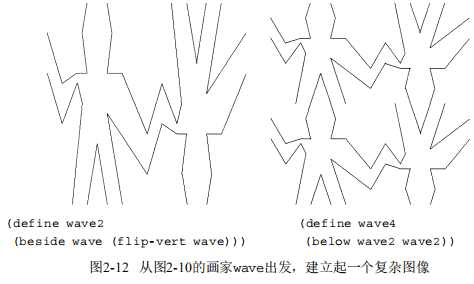(define wave2 (beside wave (flip-vert wave)))
(define wave4 (below wave2 wave2))

(define (flipped-pairs painter)
(let ((painter2 (beside painter (flip-vert painter))))
(below painter2 painter2)))

(define wave4 (flipped-pairs wave))

(define (right-split painter n)
(if (= n 0)
painter
(let ((smaller (right-split painter (- n 1))))
(beside painter (below smaller smaller)))))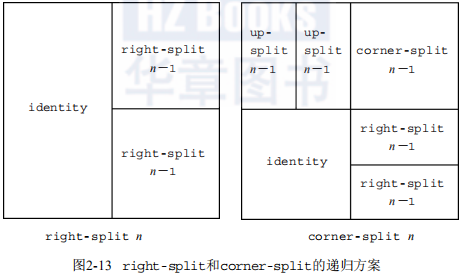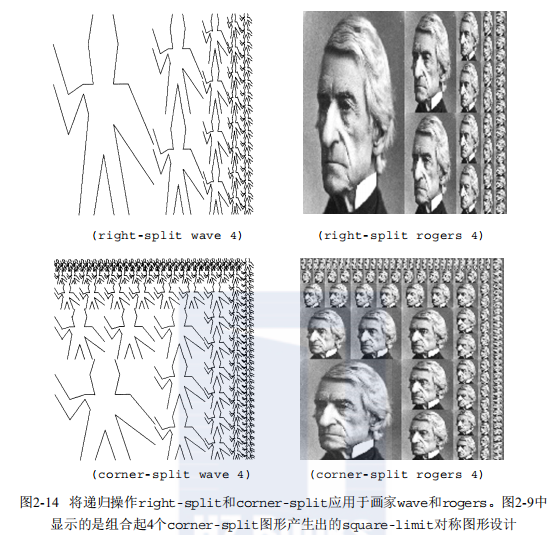(define (corner-split painter n)
(if (= n 0)
painter
(let ((up (up-split painter (- n 1)))
(right (right-split painter (- n 1))))
(let ((top-left (beside up up))
(bottom-right (below right right))
(corner (corner-split painter (- n 1))))
(beside (below painter top-left)
(below bottom-right corner))))))

(define (square-limit painter n)
(let ((quarter (corner-split painter n)))
(let ((half (beside (flip-horiz quarter) quarter)))
(below (flip-vert half) half))))

(define (square-of-four tl tr bl br)
(lambda (painter)
(let ((top (beside (tl painter) (tr painter)))
(bottom (beside (bl painter) (br painter))))
(below bottom top))))

(define (flipped-pairs painter)
(let ((combine4 (square-of-four identity flip-vert
identity flip-vert)))
(combine4 painter)))

(define (square-limit painter n)
(let ((combine4 (square-of-four flip-horiz identity
rotate180 flip-vert)))
(combine4 (corner-split painter n))))

(define right-split (split beside below))
(define up-split (split below beside))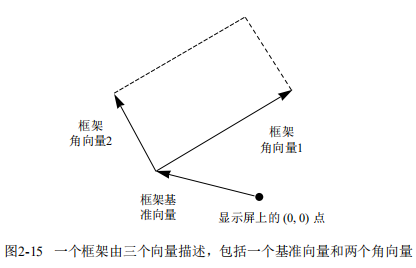Origin (Frame)＋x·Edge1 (Frame)＋y·Edge2 (Frame)

(define (frame-coord-map frame)
(lambda (v)
(origin-frame frame)
(edge1-frame frame))
(scale-vect (ycor-vect v)
(edge2-frame frame))))))

((frame-coord-map a-frame) (make-vect 0 0))

(origin-frame a-frame)

(x1，y1)＋(x2，y2)＝(x1＋x2，y1＋y2)
(x1，y1)－(x2，y2)＝(x1－x2，y1－y2)
s·(x，y)＝(sx，sy)

(define (make-frame origin edge1 edge2)
(list origin edge1 edge2))
(define (make-frame origin edge1 edge2)
(cons origin (cons edge1 edge2)))

(define (segments->painter segment-list)
(lambda (frame)
(for-each
(lambda (segment)
(draw-line
((frame-coord-map frame) (start-segment segment))
((frame-coord-map frame) (end-segment segment))))
segment-list)))

a) 画出给定框架边界的画家。
b) 通过连接框架两对角画出一个大叉子的画家。
c) 通过连接框架各边的中点画出一个菱形的画家。
d) 画家wave。

(define (transform-painter painter origin corner1 corner2)
(lambda (frame)
(let ((m (frame-coord-map frame)))
(let ((new-origin (m origin)))
(painter
(make-frame new-origin
(sub-vect (m corner1) new-origin)
(sub-vect (m corner2) new-origin)))))))

(define (flip-vert painter)
(transform-painter painter
(make-vect 0.0 1.0)   ; new origin
(make-vect 1.0 1.0)   ; new end of edge1
(make-vect 0.0 0.0))) ; new end of edge2

(define (shrink-to-upper-right painter)
(transform-painter painter
(make-vect 0.5 0.5)
(make-vect 1.0 0.5)
(make-vect 0.5 1.0)))

(define (rotate90 painter)
(transform-painter painter
(make-vect 1.0 0.0)
(make-vect 1.0 1.0)
(make-vect 0.0 0.0)))

(define (squash-inwards painter)
(transform-painter painter
(make-vect 0.0 0.0)
(make-vect 0.65 0.35)
(make-vect 0.35 0.65)))

(define (beside painter1 painter2)
(let ((split-point (make-vect 0.5 0.0)))
(let ((paint-left
(transform-painter painter1
(make-vect 0.0 0.0)
split-point
(make-vect 0.0 1.0)))
(paint-right
(transform-painter painter2
split-point
(make-vect 1.0 0.0)
(make-vect 0.5 1.0))))
(lambda (frame)
(paint-left frame)
(paint-right frame)))))

a) 给练习2.49的基本wave画家加入某些线段（例如，加上一个笑脸）。
b) 修改corner-split的构造模式（例如，只用up-split和right-split的图像的各一个副本，而不是两个）。
c) 修改square-limit，换一种使用square-of-four的方式，以另一种不同模式组合起各个角区（例如，你可以让大的Rogers先生从正方形的每个角向外看）。

## 2.3 符号数据

### 2.3.1 引号

(a b c d)
(23 45 17)
((Norah 12) (Molly 9) (Anna 7) (Lauren 6) (Charlotte 4))

(* (+ 23 45) (+ x 9))
(define (fact n) (if (= n 1) 1 (* n (fact (- n 1)))))

(define a 1)
(define b 2)
(list a b)
(1 2)
(list 'a 'b)
(a b)
(list 'a b)
(a 2)

(car 'a b c))
a
(cdr 'a b c))
(b c)

(define (memq item x)
(cond ((null? x) false)
((eq? item (car x)) x)
(else (memq item (cdr x)))))

(memq 'apple '(pear banana prune))

(memq 'apple '(x (apple sauce) y apple pear))

(list 'a 'b 'c)
(list (list 'george))
(cdr '(x1 x2) (y1 y2)))
(pair? (car ?a short list)))
(memq 'red '(red shoes) (blue socks)))
(memq 'red 'red shoes blue socks))

(equal? '(this is a list) '(this is a list))

(equal? '(this is a list) '(this (is a) list))

(car ''abracadabra)

### 2.3.2 实例：符号求导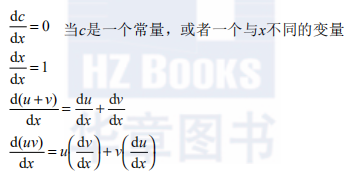(variable? e)     e是变量吗？
(same-variable? v1 v2)     v1和v2是同一个变量吗？
(sum? e)     e是和式吗？
(augend e)     e的加数
(make-sum a1 a2)     构造起a1与a2的和式
(product? e)     e是乘式吗？
(multiplier e)     e的被乘数
(multiplicand e)     e的乘数
(make-product m1 m2)     构造起m1与m2的乘式

(define (deriv exp var)
(cond ((number? exp) 0)
((variable? exp)
(if (same-variable? exp var) 1 0))
((sum? exp)
(deriv (augend exp) var)))
((product? exp)
(make-sum
(make-product (multiplier exp)
(deriv (multiplicand exp) var))
(make-product (deriv (multiplier exp) var)
(multiplicand exp))))
(else
(error "unknown expression type -- DERIV" exp))))

• 变量就是符号，它们可以用基本谓词symbol?判断：
(define (variable? x) (symbol? x))
• 两个变量相同就是表示它们的符号相互eq?：
(define (same-variable? v1 v2)
(and (variable? v1) (variable? v2) (eq? v1 v2)))
• 和式与乘式都构造为表：
(define (make-sum a1 a2) (list ? a1 a2))
(define (make-product m1 m2) (list ? m1 m2))
• 和式就是第一个元素为符号＋的表：
(define (sum? x)
(and (pair? x) (eq? (car x) '()))
• 被加数是表示和式的表里的第二个元素：
(define (addend s) (cadr s))
• 加数是表示和式的表里的第三个元素：
(define (augend s) (caddr s))
• 乘式就是第一个元素为符号 * 的表：
(define (product? x)
(and (pair? x) (eq? (car x) '()))
• 被乘数是表示乘式的表里的第二个元素：
(define (multiplier p) (cadr p))
• 乘数是表示乘式的表里的第三个元素：
(define (multiplicand p) (caddr p))

(deriv '(+ x 3) 'x)
(+ 1 0)
(deriv '(* x y) 'x)
(+ (* x 0) (* 1 y))
(deriv '(* (* x y) (+ x 3)) 'x)
(+ (* (* x y) (+ 1 0))
(* (+ (* x 0) (* 1 y))
(+  x 3)))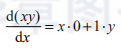(define (make-sum a1 a2)
(cond ((=number? a1 0) a2)
((=number? a2 0) a1)
((and (number? a1) (number? a2)) (+ a1 a2))
(else (list ? a1 a2))))

(define (=number? exp num)
(and (number? exp) (= exp num)))

(define (make-product m1 m2)
(cond ((or (=number? m1 0) (=number? m2 0)) 0)
((=number? m1 1) m2)
((=number? m2 1) m1)
((and (number? m1) (number? m2)) (* m1 m2))
(else (list ? m1 m2))))

(deriv '(+ x 3) 'x)
1
(deriv '(* x y) 'x)
y
(deriv '(* (* x y) (+ x 3)) 'x)
(+ (* x y) (* y (+ x 3)))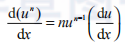(deriv '(* x y (+ x 3)) 'x)

a) 请说明怎样做出这些过程，以便完成在中缀表示形式（例如（x＋（3（x＋（y＋2）））））上的代数表达式求导。为了简化有关的工作，现在可以假定＋和 总是取两个参数，而且表达式中已经加上了所有的括号。
b) 如果允许标准的代数写法，例如（x ＋ 3 *（x ＋ y ＋ 2）），问题就会变得更困难许多。在这种表达式里可能不写不必要的括号，并要假定乘法应该在加法之前完成。你还能为这种表示方式设计好适当的谓词、选择函数和构造函数，使我们的求导程序仍然能工作吗？

### 2.3.3 实例：集合的表示

(define (element-of-set? x set)
(cond ((null? set) false)
((equal? x (car set)) true)
(else (element-of-set? x (cdr set)))))

(define (adjoin-set x set)
(if (element-of-set? x set)
set
(cons x set)))

(define (intersection-set set1 set2)
(cond ((or (null? set1) (null? set2)) '())
((element-of-set? (car set1) set2)
(cons (car set1)
(intersection-set (cdr set1) set2)))
(else (intersection-set (cdr set1) set2))))

(define (element-of-set? x set)
(cond ((null? set) false)
((= x (car set)) true)
((< x (car set)) false)
(else (element-of-set? x (cdr set)))))

(define (intersection-set set1 set2)
(if (or (null? set1) (null? set2))
'()
(let ((x1 (car set1)) (x2 (car set2)))
(cond ((= x1 x2)
(cons x1
(intersection-set (cdr set1)
(cdr set2))))
((< x1 x2)
(intersection-set (cdr set1) set2))
((< x2 x1)
(intersection-set set1 (cdr set2)))))))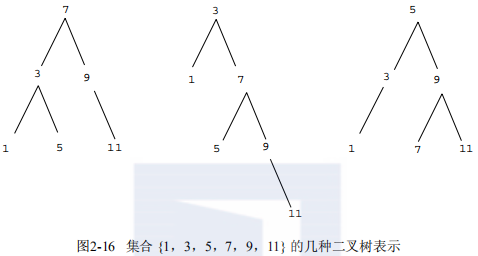(define (entry tree) (car tree))
(define (make-tree entry left right)
(list entry left right))

(define (element-of-set? x set)
(cond ((null? set) false)
((= x (entry set)) true)
((< x (entry set))
(element-of-set? x (left-branch set)))
((> x (entry set))
(element-of-set? x (right-branch set)))))

(define (adjoin-set x set)
(cond ((null? set) (make-tree x '() '()))
((= x (entry set)) set)
((< x (entry set))
(make-tree (entry set)
(right-branch set)))
((> x (entry set))
(make-tree (entry set)
(left-branch set)
(adjoin-set x (right-branch set))))))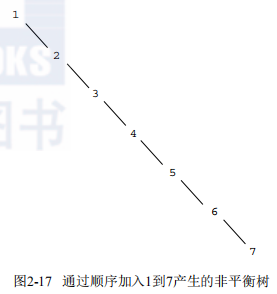(define (tree->list-1 tree)
(if (null? tree)
'()
(append (tree->list-1 (left-branch tree))
(cons (entry tree)
(tree->list-1 (right-branch tree))))))
(define (tree->list-2 tree)
(define (copy-to-list tree result-list)
(if (null? tree)
result-list
(copy-to-list (left-branch tree)
(cons (entry tree)
(copy-to-list (right-branch tree)
result-list)))))
(copy-to-list tree '()))

a) 这两个过程对所有的树都产生同样结果吗？如果不是，它们产生出的结果有什么不同？它们对图2-16中的那些树产生什么样的表？
b) 将n个结点的平衡树变换为表时，这两个过程所需的步数具有同样量级的增长速度吗？如果不一样，哪个过程增长得慢一些？

(define (list->tree elements)
(car (partial-tree elements (length elements))))
(define (partial-tree elts n)
(if (= n 0)
(cons '() elts)
(let ((left-size (quotient (- n 1) 2)))
(let ((left-result (partial-tree elts left-size)))
(let ((left-tree (car left-result))
(non-left-elts (cdr left-result))
(right-size (- n (+ left-size 1))))
(let ((this-entry (car non-left-elts))
(right-result (partial-tree (cdr non-left-elts)
right-size)))
(let ((right-tree (car right-result))
(remaining-elts (cdr right-result)))
(cons (make-tree this-entry left-tree right-tree)
remaining-elts))))))))

a) 请简要地并尽可能清楚地解释为什么partial-tree能完成工作。请画出将list->tree用于表（1 3 5 7 9 11）产生出的树。
b) 过程list->tree转换n个元素的表所需的步数以什么量级增长？

(define (lookup given-key set-of-records)
(cond ((null? set-of-records) false)
((equal? given-key (key (car set-of-records)))
(car set-of-records))
(else (lookup given-key (cdr set-of-records)))))

### 2.3.4 实例：Huffman编码树

A 000     C 010     E 100     G 110
B 001     D 011     F 101     H 111

001000010000011000100000101000001001000000000110000111

A 0     C 1010     E 1100     G 1110
B 100     D 1011     F 1101     H 1111

100010100101101100011010100100000111001111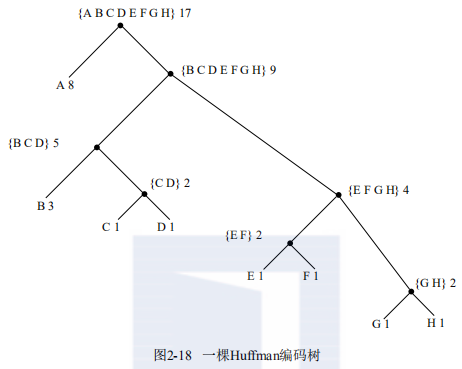初始树叶    {(A 8) (B 3) (C 1) (D 1) (E 1) (F 1) (G 1) (H 1)}

Huffman树的表示

(define (make-leaf symbol weight)
(list 'leaf symbol weight))
(define (leaf? object)
(eq? (car object) 'leaf))
(define (weight-leaf x) (caddr x))

(define (make-code-tree left right)
(list left
right
(append (symbols left) (symbols right))
(+ (weight left) (weight right))))

(define (left-branch tree) (car tree))
(define (symbols tree)
(if (leaf? tree)
(list (symbol-leaf tree))
(define (weight tree)
(if (leaf? tree)
(weight-leaf tree)
(cadddr tree)))

(define (decode bits tree)
(define (decode-1 bits current-branch)
(if (null? bits)
'()
(let ((next-branch
(choose-branch (car bits) current-branch)))
(if (leaf? next-branch)
(cons (symbol-leaf next-branch)
(decode-1 (cdr bits) tree))
(decode-1 (cdr bits) next-branch)))))
(decode-1 bits tree))
(define (choose-branch bit branch)
(cond ((= bit 0) (left-branch branch))
((= bit 1) (right-branch branch))
(else (error "bad bit -- CHOOSE-BRANCH" bit))))

(define (adjoin-set x set)
(cond ((null? set) (list x))
((< (weight x) (weight (car set))) (cons x set))
(else (cons (car set)
(adjoin-set x (cdr set))))))

(define (make-leaf-set pairs)
(if (null? pairs)
'()
(let ((pair (car pairs)))
(adjoin-set (make-leaf (car pair)    ; symbol
(make-leaf-set (cdr pairs))))))

(define sample-tree
(make-code-tree (make-leaf 誂 4)
(make-code-tree
(make-leaf 誃 2)
(make-code-tree (make-leaf 誅 1)
(make-leaf 誄 1)))))
(define sample-message ?0 1 1 0 0 1 0 1 0 1 1 1 0))

(define (encode message tree)
(if (null? message)
'()
(append (encode-symbol (car message) tree)
(encode (cdr message) tree))))

(define (generate-huffman-tree pairs)
(successive-merge (make-leaf-set pairs)))

A     2     NA     16
BOOM     1     SHA     3
GET     2     YIP     9
JOB     2     WAH     1

Get a job
Sha na na na na na na na na
Get a job
Sha na na na na na na na na
Wah yip yip yip yip yip yip yip yip yip
Sha boom

## 2.4 抽象数据的多重表示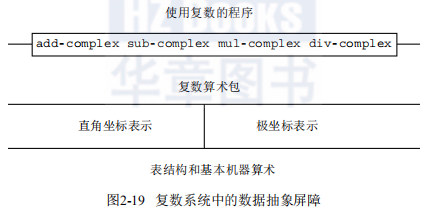### 2.4.1 复数的表示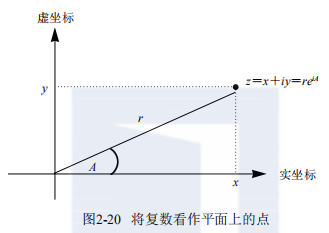(make-from-real-imag (real-part z) (imag-part z))

(make-from-mag-ang (magnitude z) (angle z))

(define (add-complex z1 z2)
(make-from-real-imag (+ (real-part z1) (real-part z2))
(+ (imag-part z1) (imag-part z2))))
(define (sub-complex z1 z2)
(make-from-real-imag (- (real-part z1) (real-part z2))
(- (imag-part z1) (imag-part z2))))
(define (mul-complex z1 z2)
(make-from-mag-ang (* (magnitude z1) (magnitude z2))
(+ (angle z1) (angle z2))))
(define (div-complex z1 z2)
(make-from-mag-ang (/ (magnitude z1) (magnitude z2))
(- (angle z1) (angle z2))))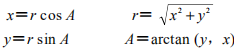(define (real-part z) (car z))
(define (imag-part z) (cdr z))
(define (magnitude z)
(sqrt (+ (square (real-part z)) (square (imag-part z)))))
(define (angle z)
(atan (imag-part z) (real-part z)))
(define (make-from-real-imag x y) (cons x y))
(define (make-from-mag-ang r a)
(cons (* r (cos a)) (* r (sin a))))

(define (real-part z)
(* (magnitude z) (cos (angle z))))
(define (imag-part z)
(* (magnitude z) (sin (angle z))))
(define (magnitude z) (car z))
(define (angle z) (cdr z))
(define (make-from-real-imag x y)
(cons (sqrt (+ (square x) (square y)))
(atan y x)))
(define (make-from-mag-ang r a) (cons r a))

### 2.4.2 带标志数据

(define (attach-tag type-tag contents)
(cons type-tag contents))
(define (type-tag datum)
(if (pair? datum)
(car datum)
(error "Bad tagged datum -- TYPE-TAG" datum)))
(define (contents datum)
(if (pair? datum)
(cdr datum)
(error "Bad tagged datum -- CONTENTS" datum)))

(define (rectangular? z)
(eq? (type-tag z) 'rectangular))
(define (polar? z)
(eq? (type-tag z) 'polar))

(define (real-part-rectangular z) (car z))
(define (imag-part-rectangular z) (cdr z))
(define (magnitude-rectangular z)
(sqrt (+ (square (real-part-rectangular z))
(square (imag-part-rectangular z)))))
(define (angle-rectangular z)
(atan (imag-part-rectangular z)
(real-part-rectangular z)))
(define (make-from-real-imag-rectangular x y)
(attach-tag 'rectangular (cons x y)))
(define (make-from-mag-ang-rectangular r a)
(attach-tag 'rectangular
(cons (* r (cos a)) (* r (sin a)))))

(define (real-part-polar z)
(* (magnitude-polar z) (cos (angle-polar z))))
(define (imag-part-polar z)
(* (magnitude-polar z) (sin (angle-polar z))))
(define (magnitude-polar z) (car z))
(define (angle-polar z) (cdr z))
(define (make-from-real-imag-polar x y)
(attach-tag 'polar
(cons (sqrt (+ (square x) (square y)))
(atan y x))))
(define (make-from-mag-ang-polar r a)
(attach-tag 'polar (cons r a)))

(define (real-part z)
(cond ((rectangular? z)
(real-part-rectangular (contents z)))
((polar? z)
(real-part-polar (contents z)))
(else (error "Unknown type -- REAL-PART" z))))
(define (imag-part z)
(cond ((rectangular? z)
(imag-part-rectangular (contents z)))
((polar? z)
(imag-part-polar (contents z)))
(else (error "Unknown type -- IMAG-PART" z))))
(define (magnitude z)
(cond ((rectangular? z)
(magnitude-rectangular (contents z)))
((polar? z)
(magnitude-polar (contents z)))
(else (error "Unknown type -- MAGNITUDE" z))))
(define (angle z)
(cond ((rectangular? z)
(angle-rectangular (contents z)))
((polar? z)
(angle-polar (contents z)))
(else (error "Unknown type -- ANGLE" z))))

(define (add-complex z1 z2)
(make-from-real-imag (+ (real-part z1) (real-part z2))
(+ (imag-part z1) (imag-part z2))))

(define (make-from-real-imag x y)
(make-from-real-imag-rectangular x y))
(define (make-from-mag-ang r a)
(make-from-mag-ang-polar r a))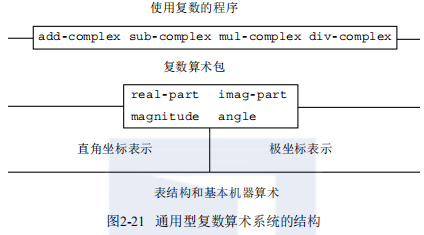### 2.4.3 数据导向的程序设计和可加性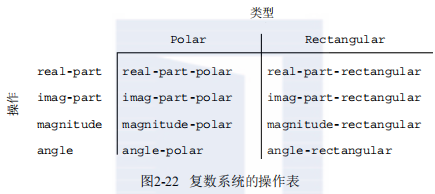• (put <op> <type> <item>)

• (get <op> <type>)

(define (install-rectangular-package)
;; internal procedures
(define (real-part z) (car z))
(define (imag-part z) (cdr z))
(define (make-from-real-imag x y) (cons x y))
(define (magnitude z)
(sqrt (+ (square (real-part z))
(square (imag-part z)))))
(define (angle z)
(atan (imag-part z) (real-part z)))
(define (make-from-mag-ang r a)
(cons (* r (cos a)) (* r (sin a))))
;; interface to the rest of the system
(define (tag x) (attach-tag 'rectangular x))
(put 'real-part ?rectangular) real-part)
(put 'imag-part ?rectangular) imag-part)
(put 'magnitude ?rectangular) magnitude)
(put 'angle ?rectangular) angle)
(put 'make-from-real-imag 'rectangular
(lambda (x y) (tag (make-from-real-imag x y))))
(put 'make-from-mag-ang 'rectangular
(lambda (r a) (tag (make-from-mag-ang r a))))
'done)

Alyssa的极坐标包与此类似：

(define (install-polar-package)
;; internal procedures
(define (magnitude z) (car z))
(define (angle z) (cdr z))
(define (make-from-mag-ang r a) (cons r a))
(define (real-part z)
(* (magnitude z) (cos (angle z))))
(define (imag-part z)
(* (magnitude z) (sin (angle z))))
(define (make-from-real-imag x y)
(cons (sqrt (+ (square x) (square y)))
(atan y x)))
;; interface to the rest of the system
(define (tag x) (attach-tag 'polar x))
(put 'real-part ?polar) real-part)
(put 'imag-part ?polar) imag-part)
(put 'magnitude ?polar) magnitude)
(put 'angle ?polar) angle)
(put 'make-from-real-imag 'polar
(lambda (x y) (tag (make-from-real-imag x y))))
(put 'make-from-mag-ang 'polar
(lambda (r a) (tag (make-from-mag-ang r a))))
'done)

(define (apply-generic op . args)
(let ((type-tags (map type-tag args)))
(let ((proc (get op type-tags)))
(if proc
(apply proc (map contents args))
(error
"No method for these types -- APPLY-GENERIC"
(list op type-tags))))))

(define (real-part z) (apply-generic 'real-part z))
(define (imag-part z) (apply-generic 'imag-part z))
(define (magnitude z) (apply-generic 'magnitude z))
(define (angle z) (apply-generic 'angle z))

(define (make-from-real-imag x y)
((get 'make-from-real-imag 'rectangular) x y))
(define (make-from-mag-ang r a)
((get 'make-from-mag-ang 'polar) r a))

(define (deriv exp var)
(cond ((number? exp) 0)
((variable? exp) (if (same-variable? exp var) 1 0))
((sum? exp)
(deriv (augend exp) var)))
((product? exp)
(make-sum
(make-product (multiplier exp)
(deriv (multiplicand exp) var))
(make-product (deriv (multiplier exp) var)
(multiplicand exp))))
<更多规则可以加在这里>
(else (error "unknown expression type -- DERIV" exp))))

(define (deriv exp var)
(cond ((number? exp) 0)
((variable? exp) (if (same-variable? exp var) 1 0))
(else ((get 'deriv (operator exp)) (operands exp)
var))))
(define (operator exp) (car exp))
(define (operands exp) (cdr exp))

a) 请解释上面究竟做了些什么。为什么我们无法将相近的谓词number?和same-variable?也加入数据导向分派中？
b) 请写出针对和式与积式的求导过程，并把它们安装到表格里，以便上面程序使用所需要的辅助性代码。
c) 请选择一些你希望包括的求导规则，例如对乘幂（练习2.56）求导等等，并将它们安装到这一数据导向的系统里。
d) 在这一简单的代数运算器中，表达式的类型就是构造起它们来的代数运算符。假定我们想以另一种相反的方式做索引，使得deriv里完成分派的代码行像下面这样：

((get (operator exp) 'deriv) (operands exp) var)

a) 请为公司总部实现一个get-record过程，使它能从一个特定的人事文件里提取出一个特定的雇员记录。这一过程应该能应用于任何分支机构的文件。请说明各个独立分支机构的文件应具有怎样的结构。特别是考虑，它们必须提供哪些类型信息？
b) 请为公司总部实现一个get-salary过程，它能从任何分支机构的人事文件中取得某个给定雇员的薪金信息。为了使这一操作能够工作，这些记录应具有怎样的结构？
c) 请为公司总部实现一个过程find-employee-record，该过程需要针对一个特定雇员名，在所有分支机构的文件去查找对应的记录，并返回找到的记录。假定这一过程的参数是一个雇员名和所有分支文件的表。
d) 当Insatiable购并新公司后，要将新的人事文件结合到系统中，必须做哪些修改？

(define (make-from-real-imag x y)
(define (dispatch op)
(cond ((eq? op 'real-part) x)
((eq? op 'imag-part) y)
((eq? op 'magnitude)
(sqrt (+ (square x) (square y))))
((eq? op 'angle) (atan y x))
(else
(error "Unknown op -- MAKE-FROM-REAL-IMAG" op))))
dispatch)

(define (apply-generic op arg) (arg op))

## 2.5 带有通用型操作的系统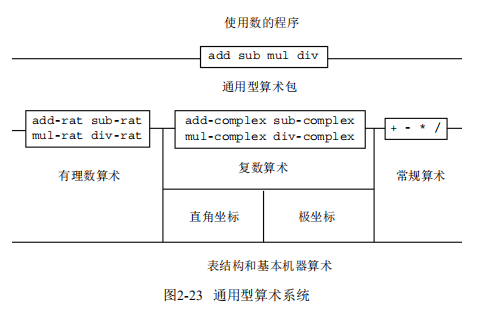### 2.5.1 通用型算术运算

(define (add x y) (apply-generic 'add x y))
(define (sub x y) (apply-generic 'aub x y))
(define (mul x y) (apply-generic 'mul x y))
(define (div x y) (apply-generic 'div x y))

(define (install-scheme-number-package)
(define (tag x)
(attach-tag 'acheme-number x))
(lambda (x y) (tag (+ x y))))
(put 'aub ?scheme-number scheme-number)
(lambda (x y) (tag (- x y))))
(put 'mul ?scheme-number scheme-number)
(lambda (x y) (tag (* x y))))
(put 'div ?scheme-number scheme-number)
(lambda (x y) (tag (/ x y))))
(put 'make 'acheme-number
(lambda (x) (tag x)))
'done)

Scheme数值包的用户可以通过下面过程，创建带标志的常规数：

(define (make-scheme-number n)
((get 'make 'acheme-number) n))

(define (install-rational-package)
;; internal procedures
(define (numer x) (car x))
(define (denom x) (cdr x))
(define (make-rat n d)
(let ((g (gcd n d)))
(cons (/ n g) (/ d g))))
(make-rat (+ (* (numer x) (denom y))
(* (numer y) (denom x)))
(* (denom x) (denom y))))
(define (sub-rat x y)
(make-rat (- (* (numer x) (denom y))
(* (numer y) (denom x)))
(* (denom x) (denom y))))
(define (mul-rat x y)
(make-rat (* (numer x) (numer y))
(* (denom x) (denom y))))
(define (div-rat x y)
(make-rat (* (numer x) (denom y))
(* (denom x) (numer y))))
;; interface to rest of the system
(define (tag x) (attach-tag 'rational x))
(lambda (x y) (tag (add-rat x y))))
(put 'aub ?rational rational)
(lambda (x y) (tag (sub-rat x y))))
(put 'mul ?rational rational)
(lambda (x y) (tag (mul-rat x y))))
(put 'div ?rational rational)
(lambda (x y) (tag (div-rat x y))))
(put 'make 'rational
(lambda (n d) (tag (make-rat n d))))
'done)
(define (make-rational n d)
((get 'make 'rational) n d))

(define (install-complex-package)
;; imported procedures from rectangular and polar packages
(define (make-from-real-imag x y)
((get 'make-from-real-imag 'rectangular) x y))
(define (make-from-mag-ang r a)
((get 'make-from-mag-ang 'polar) r a))
;; internal procedures
(make-from-real-imag (+ (real-part z1) (real-part z2))
(+ (imag-part z1) (imag-part z2))))
(define (sub-complex z1 z2)
(make-from-real-imag (- (real-part z1) (real-part z2))
(- (imag-part z1) (imag-part z2))))
(define (mul-complex z1 z2)
(make-from-mag-ang (* (magnitude z1) (magnitude z2))
(+ (angle z1) (angle z2))))
(define (div-complex z1 z2)
(make-from-mag-ang (/ (magnitude z1) (magnitude z2))
(- (angle z1) (angle z2))))
;; interface to rest of the system
(define (tag z) (attach-tag 'complex z))
(lambda (z1 z2) (tag (add-complex z1 z2))))
(put 'aub ?complex complex)
(lambda (z1 z2) (tag (sub-complex z1 z2))))
(put 'mul ?complex complex)
(lambda (z1 z2) (tag (mul-complex z1 z2))))
(put 'div ?complex complex)
(lambda (z1 z2) (tag (div-complex z1 z2))))
(put 'make-from-real-imag 'complex
(lambda (x y) (tag (make-from-real-imag x y))))
(put 'make-from-mag-ang 'complex
(lambda (r a) (tag (make-from-mag-ang r a))))
'done)

(define (make-complex-from-real-imag x y)
((get 'make-from-real-imag 'complex) x y))
(define (make-complex-from-mag-ang r a)
((get 'make-from-mag-ang 'complex) r a))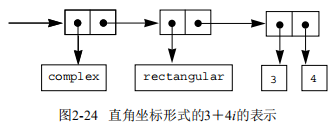(put 'real-part '(complex) real-part)
(put 'imag-part '(complex) imag-part)
(put 'magnitude '(complex) magnitude)
(put 'angle '(complex) angle)

### 2.5.2 不同类型数据的组合

;; to be included in the complex package
(make-from-real-imag (+ (real-part z) x)
(imag-part z)))
(lambda (z x) (tag (add-complex-to-schemenum z x))))

(define (scheme-number->complex n)
(make-complex-from-real-imag (contents n) 0))

(put-coercion 'acheme-number 'complex scheme-number->complex)

（这里假定了存在着用于操纵这个表格的put-coercion和get-coercion过程。）一般而言，这一表格里的某些格子将是空的，因为将任何数据对象转换到另一个类型并不是都能做的。例如并不存在某种将任意复数转换为常规数值的方式，因此，这个表格中就不应包括一般性的complex->scheme-number过程。

(define (apply-generic op . args)
(let ((type-tags (map type-tag args)))
(let ((proc (get op type-tags)))
(if proc
(apply proc (map contents args))
(if (= (length args) 2)
(let ((type1 (car type-tags))
(a1 (car args))
(let ((t1->t2 (get-coercion type1 type2))
(t2->t1 (get-coercion type2 type1)))
(cond (t1->t2
(apply-generic op (t1->t2 a1) a2))
(t2->t1
(apply-generic op a1 (t2->t1 a2)))
(else
(error "No method for these types"
(list op type-tags))))))
(error "No method for these types"
(list op type-tags)))))))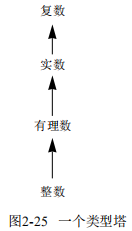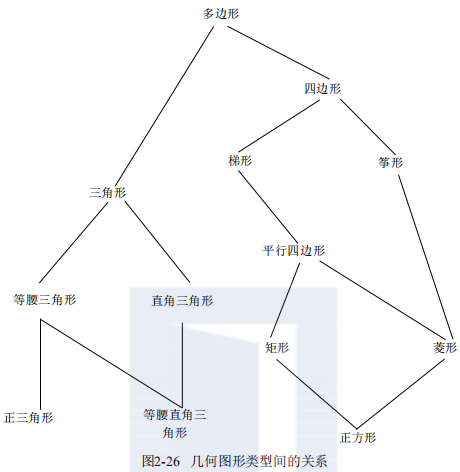(define (scheme-number->scheme-number n) n)
(define (complex->complex z) z)
(put-coercion 'acheme-number 'acheme-number
scheme-number->scheme-number)
(put-coercion 'complex 'complex complex->complex)

a) 如果安装了Louis的强制过程，如果在调用apply-generic时各参数的类型都为scheme-number或者类型都为complex，而在表格中又找不到相应的操作，这时会出现什么情况？例如，假定我们定义了一个通用型的求幂运算：

(define (exp x y) (apply-generic 'exp x y))

;; following added to Scheme-number package
(put 'exp ?scheme-number scheme-number)
(lambda (x y) (tag (expt x y)))) ; using primitive expt

b) Louis真的纠正了有关同样类型参数的强制问题吗？apply-generic还能像原来那样正确工作吗？
c) 请修改apply-generic，使之不会试着去强制两个同样类型的参数。

### 2.5.3 实例：符号代数

x2 sin (y2＋1)＋x cos 2y＋cos (y3－2y2)

5x2＋3x＋7

(y2＋1) x3＋(2y) x＋1

define (add-poly p1 p2)
(if (same-variable? (variable p1) (variable p2))
(make-poly (variable p1)
(term-list p2)))
(error "Polys not in same var -- ADD-POLY"
(list p1 p2))))
(define (mul-poly p1 p2)
(if (same-variable? (variable p1) (variable p2))
(make-poly (variable p1)
(mul-terms (term-list p1)
(term-list p2)))
(error "Polys not in same var -- MUL-POLY"
(list p1 p2))))

(define (install-polynomial-package)
;; internal procedures
;; representation of poly
(define (make-poly variable term-list)
(cons variable term-list))
(define (variable p) (car p))
(define (term-list p) (cdr p))
<过程 same-variable? 和 variable? 取自2.3.2节>
;; representation of terms and term lists
(define (mul-poly p1 p2) ...)
<mul-poly 使用的过程>
;; interface to rest of the system
(define (tag p) (attach-tag 'polynomial p))
(lambda (p1 p2) (tag (add-poly p1 p2))))
(put 'mul ?polynomial polynomial)
(lambda (p1 p2) (tag (mul-poly p1 p2))))
(put 'make 'polynomial
(lambda (var terms) (tag (make-poly var terms))))
'done)

(define (add-terms L1 L2)
(cond ((empty-termlist? L1) L2)
((empty-termlist? L2) L1)
(else
(let ((t1 (first-term L1)) (t2 (first-term L2)))
(cond ((> (order t1) (order t2))
((< (order t1) (order t2))
(else
(make-term (order t1)
(rest-terms L2)))))))))

(define (mul-terms L1 L2)
(if (empty-termlist? L1)
(the-empty-termlist)
(mul-terms (rest-terms L1) L2))))
(define (mul-term-by-all-terms t1 L)
(if (empty-termlist? L)
(the-empty-termlist)
(let ((t2 (first-term L)))
(make-term (+ (order t1) (order t2))
(mul (coeff t1) (coeff t2)))
(mul-term-by-all-terms t1 (rest-terms L))))))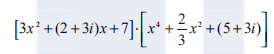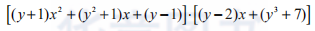A :   x5＋2x4＋3x2－2x－5

B :   x100＋2x2＋1

(define (adjoin-term term term-list)
(if (=zero? (coeff term))
term-list
(cons term term-list)))
(define (the-empty-termlist) '())
(define (first-term term-list) (car term-list))
(define (rest-terms term-list) (cdr term-list))
(define (empty-termlist? term-list) (null? term-list))
(define (make-term order coeff) (list order coeff))
(define (order term) (car term))
(define (coeff term) (cadr term))

(define (make-polynomial var terms)
((get 'make 'polynomial) var terms))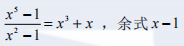(define (div-terms L1 L2)
(if (empty-termlist? L1)
(list (the-empty-termlist) (the-empty-termlist))
(let ((t1 (first-term L1))
(t2 (first-term L2)))
(if (> (order t2) (order t1))
(list (the-empty-termlist) L1)
(let ((new-c (div (coeff t1) (coeff t2)))
(new-o (- (order t1) (order t2))))
(let ((rest-of-result
<递归地计算结果的其余部分>
))
<形成完整的结果>
))))))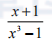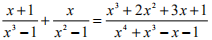（这里的和已经经过了简化，删除了公因子。常规的“交叉乘法”得到的将是一个4次多项式的分子和5次多项式的分母。）

(define p1 (make-polynomial 'x ?(2 1)(0 1))))
(define p2 (make-polynomial 'x ?(3 1)(0 1))))
(define rf (make-rational p2 p1))

(define (gcd a b)
(if (= b 0)
a
(gcd b (remainder a b))))

(define (gcd-terms a b)
(if (empty-termlist? b)
a
(gcd-terms b (remainder-terms a b))))

(define p1 (make-polynomial 'x ?(4 1) (3 -1) (2 -2) (1 2))))
(define p2 (make-polynomial 'x ?(3 1) (1 -1))))
(greatest-common-divisor p1 p2)

P1 : x2－2x＋1 P2 : 11x2＋7 P3 : 13x＋5

a) 请实现过程pseudoremainder-terms，它就像是remainder-terms，但是像上面所描述的那样，在调用div-terms之前，先将被除式乘了整数化因子。请修改gcd-terms使之能使用pseudoremainder-terms，并检验现在greatest-common-divisor能否对练习2.95的例子产生出一个整系数的答案。
b) 现在的GCD保证能得到整系数，但它们将比P1的系数大，请修改gcd-terms使它能从答案的所有系数中删除公因子，方法是将这些系数都除以它们的（整数）最大公约数。

• 用取自练习2.96的gcd-terms版本计算出分子和分母的GCD；
• 在你得到了这个GCD后，在用GCD去除分子和分母之前，先将它们都乘以同一个整数化因子，以使除以这个GCD不会引进任何非整数系数。作为这个因子，你可以使用得到的GCD的首项系数的1＋O1－O2次幂。其中O2是这个GCD的次数，O1是分子与分母的次数中大的那一个。这将保证用这个GCD去除分子和分母不会引进任何分数。
• 这一操作得到的结果将是具有整系数的分子和分母。它们的系数通常会由于整数化因子而变得非常大。所以最后一步是去除这个多余的因子，为此需要首先计算出分子和分母中所有系数的（整数）最大公约数，而后除去这个公约数。

b) 请定义一个类似于reduce-terms的过程，它完成的工作就像是make-rat对整数做的事情：

(define (reduce-integers n d)
(let ((g (gcd n d)))
(list (/ n g) (/ d g))))

(define p1 (make-polynomial 'x ?(1 1)(0 1))))
(define p2 (make-polynomial 'x ?(3 1)(0 -1))))
(define p3 (make-polynomial 'x ?(1 1))))
(define p4 (make-polynomial 'x ?(2 1)(0 -1))))
(define rf1 (make-rational p1 p2))
(define rf2 (make-rational p3 p4))
(add rf1 rf2)

GCD计算是所有需要完成有理函数操作的系统的核心。上面所使用的算法虽然在数学上直截了当，但却异常低效。低效的部分原因在于大量的除法操作，部分在于由伪除产生的巨大的中间系数。在开发代数演算系统的领域中，一个很活跃问题就是设计计算多项式GCD的更好算法。

《PolarDB-X 动手实践》系列第一期，体验如何一键安装部署PolarDB-X。|
3月前
|

61 0
|
4月前
|
Java
ChatGPT告诉你Java内部类 vs. 组合的区别
ChatGPT告诉你Java内部类 vs. 组合的区别
41 0
|
7月前
|

53 0
|
7月前
|

c++模板的概念全新解释（二）
c++模板的概念全新解释（二）
94 0
|
7月前
|

c++模板的概念全新解释（一）
c++模板的概念全新解释（一）
143 0

75 0
|

109 0